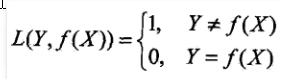# 朴素贝叶斯

hit2015spring $\frac{hit2015spring}{晨凫追风}$

### 贝叶斯与条件概率

A1,,An $A_1,\cdots,A_n$是互不相容的事件，且 P(Ai)>0(i=1,2,,n) $P(A_i)>0(i=1,2,\cdots,n)$若对任意事件 B $B$BA1+A2+,+An$B \subset A_1+A_2+\cdots,+A_n$ P(B)>0 $P(B)>0$

P(Ai|B)=P(Ai)P(B|Ai)j+1nP(Aj)P(B|Aj)

P(X=x|Y=ck)=P(X(1)=x(1),X(2)=x(2),,X(n)=x(n)|Y=ck),k=1,2,,K $P(X=x|Y=c_k)=P(X^{(1)}=x^{(1)},X^{(2)}=x^{(2)},\cdots,X^{(n)}=x^{(n)}|Y=c_k),k=1,2,\cdots,K$

P(X=x|Y=ck)=P(X(1)=x(1),X(2)=x(2),,X(n)=x(n)|Y=ck)=j=1nP(X(j)=x(j)|Y=ck) $P(X=x|Y=c_k)=P(X^{(1)}=x^{(1)},X^{(2)}=x^{(2)},\cdots,X^{(n)}=x^{(n)}|Y=c_k)=\prod \limits_{j=1}^{n}P(X^{(j)}=x^{(j)}|Y=c_k)$

P(Y=ck|X=x)=P(X=x|Y=ck)P(Y=ck)kP(X=x|Y=ck)P(Y=ck)=P(Y=ck)jP(X(j)=x(j)|Y=ck)kP(Y=ck)jP(X(j)=x(j)|Y=ck)

y=argmaxckP(Y=ck)jP(X(j)=x(j)|Y=ck)

arg $arg$表示取满足后面式子中的变量的值，即取满足 maxP(Y=ck)jP(X(j)=x(j)|Y=ck) $\max P(Y=c_k)\prod_j P(X^{(j)}=x^{(j)}|Y=c_k)$这个式子的变量 ck $c_k$的值。

### 贝叶斯的另外一个角度Rexp(f)=E[L(Y,f(X))] $R_{exp}(f)=E[L(Y,f(X))]$

Rexp(f)=EXk=1K[L(ck,f(X))]P(ck|X) $R_{exp}(f)=E_X\sum\limits_{k=1}^{K}[L(c_k,f(X))]P(c_k|X)$

f(x)=argminyYk=1KL(ck,y)P(ck|X=x) =argminyYk=1KP(yck|X=x) =argminyY(1P(y=ck|X=x)) =argmaxyYP(y=ck|X=x) $f(x)=arg \min\limits_{y \in \mathcal Y} \sum\limits_{k=1}^{K}L(c_k,y)P(c_k|X=x) \ \qquad =arg \min\limits_{y \in \mathcal Y} \sum\limits_{k=1}^{K}P(y\ne c_k|X=x)\ \qquad =arg \min\limits_{y \in \mathcal Y}(1-P(y= c_k|X=x))\ \qquad =arg \max\limits_{y \in \mathcal Y}P(y= c_k|X=x)$

### 参数估计

P(Data|M)=P(x1,x2,,x100|M)=P(x1|M)P(x2|M)P(x100|M)=p70(1p)30.

70p69(1p)30p7030(1p)29=0

y=argmaxckP(Y=ck)jP(X(j)=x(j)|Y=ck)

### 贝叶斯估计

P(Y=ck)=Dc+1D+c $P(Y=c_k)=\frac{D_c+1}{D+c}$

P(X(j)=x(j)|Y=ck)=Dxkjc+1Dc+k $P(X^{(j)}=x^{(j)}|Y=c_k)=\frac{D_c^{x_j^k}+1}{D_c+k}$

08-165989
07-3059606-1651
03-21455
08-16331
03-102198
09-271398
12-303491